# CBSE Class 12 Mathematics Application Of Integration Notes

Download CBSE Class 12 Mathematics Application Of Integration Notes in PDF format. All Revision notes for Class 12 Application Of Integrals have been designed as per the latest syllabus and updated chapters given in your textbook for Application Of Integrals in Standard 12. Our teachers have designed these concept notes for the benefit of Grade 12 students. You should use these chapter wise notes for revision on daily basis. These study notes can also be used for learning each chapter and its important and difficult topics or revision just before your exams to help you get better scores in upcoming examinations, You can also use Printable notes for Class 12 Application Of Integrals for faster revision of difficult topics and get higher rank. After reading these notes also refer to MCQ questions for Class 12 Application Of Integrals given our website

## Class 12 Application Of Integrals Revision Notes

Class 12 Application Of Integrals students should refer to the following concepts and notes for Application Of Integrals in standard 12. These exam notes for Grade 12 Application Of Integrals will be very useful for upcoming class tests and examinations and help you to score good marks

### Notes Class 12 Application Of Integrals

(A) KEY CONCEPTS

1. AREA LYING BELOW THE X-AXIS:

If f(x)≤0 for a≤x≤b,then the graph of y=f(x) lies below x-axis Therefore area bounded by the curve y=f(x),x-axis and the ordinates x=a and x=b is given by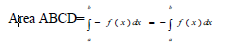2. AREA LYING ABOVE THE X-AXIS:

The area enclosed by the curve y= f(x), x-axis & between the ordinate at x=a & x=b is given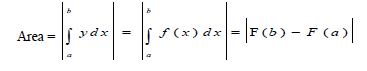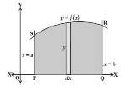3. AREA LYING ON RIGHT OF Y-AXIS :

Area bounded by the curve x=f(y),y-axis and the abscissa y=c and y=d is given by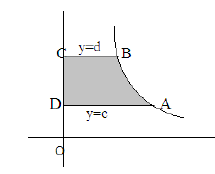4. AREA LYING ON LEFT OF Y-AXIS:

The area enclosed by the curve x= f(y), y-axis & between the abscissa at y=c & y=d is given by :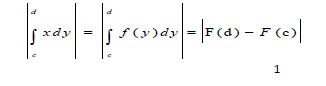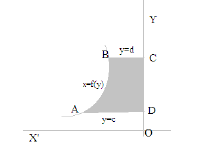5. AREA BOUNDED BY TWO CURVES

Area bounded by the two curves y = f(x) & y = g(x) where f1(x) f2(x) in a , b & between the ordinate x=a & x=b is given by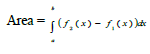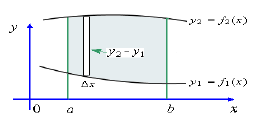IMPORTANT FORMULAE TO USE :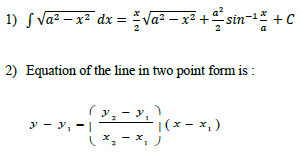Important Notes

1. If the equation of the curve contains only even powers of x, then the curve is symmetrical about y-axis

2. If the equation of the curve contains only even powers of y, then the curve is symmetrical about x-axis.

3. If the equation of the curve remains unchanged when x is replaced by x and y by y, then the curve is symmetrical in opposite quadrants.

4. If the equation of the curve remains unchanged when x and y are interchanged ,then the curve is symmetrical about the line y=x

1. Find the area of the region {(x,y):x2 ≤ y ≤ x }

Sol. The required area is bounded between two curves y =x2 and y= x . Both of these curves are symmetric about y-axis and shaded region in the fig. shows the region whose area is required.

Therefore, required area =2× area of region R1

Now to find point of intersection of curves y =x2 and y= x , we solve them simultaneously.

Clearly, region R1 is in first quadrant, where x>0

x =x => y =x…………….(i)

y =x2…………….(ii)

either x = 0 or x = 1

The limits are , when x=0, y=0 and when x=1, y=1

So points of intersection of the curve are o(0,0) and A(1,1)

Now, required area = 2× area of region R1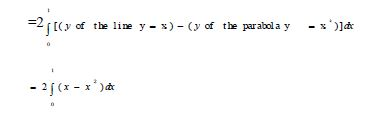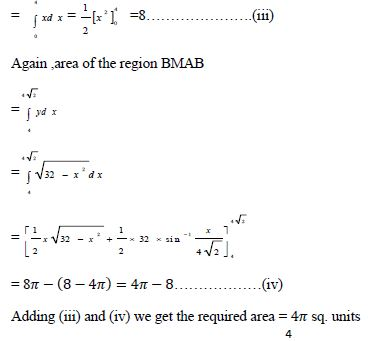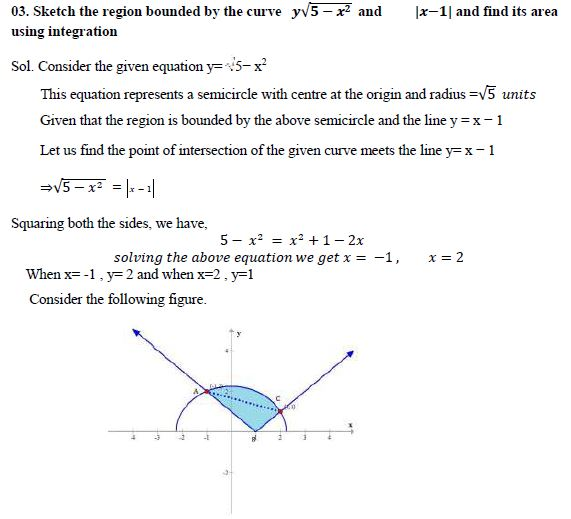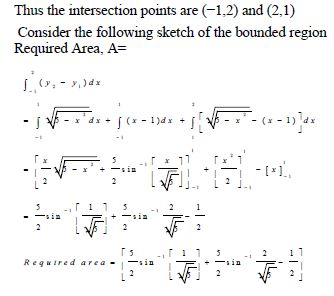## Tags:

Click for more Application Of Integrals Study Material
 CBSE Class 12 Mathematics Application Of Integration Notes

## Latest NCERT & CBSE News

Read the latest news and announcements from NCERT and CBSE below. Important updates relating to your studies which will help you to keep yourself updated with latest happenings in school level education. Keep yourself updated with all latest news and also read articles from teachers which will help you to improve your studies, increase motivation level and promote faster learning

### Heritage India Quiz 2021 2022

CBSE Heritage India Quiz is conducted every year to raise the awareness about the preserving human heritage, diversity and vulnerability of the India's built monuments and heritage sites. It is an attempt of the Board to motivate the future generations of this country...

### Celebration of Matribhasha Diwas Mother Language day

UNESCO has declared 21st February of every year to be celebrated as International Mother Language day to promote dissemination of Mother Language of all, create awareness of linguistic and cultural traditions and diversity across the world and to inspire solidarity...

This is with reference to CBSE Notification No. 40/2021 dated 04.05.2021 regarding the Innovation Ambassador program – An online training program for teachers by CBSE in collaboration with Ministry of Education’s Innovation Cell (MIC) and AICTE. In view of the current...

### Board Exams Date Sheet Class 10 and Class 12

Datesheet for CBSE Board Exams Class 10  (Scroll down for Class 12 Datesheet) Datesheet for CBSE Board Exams Class 12